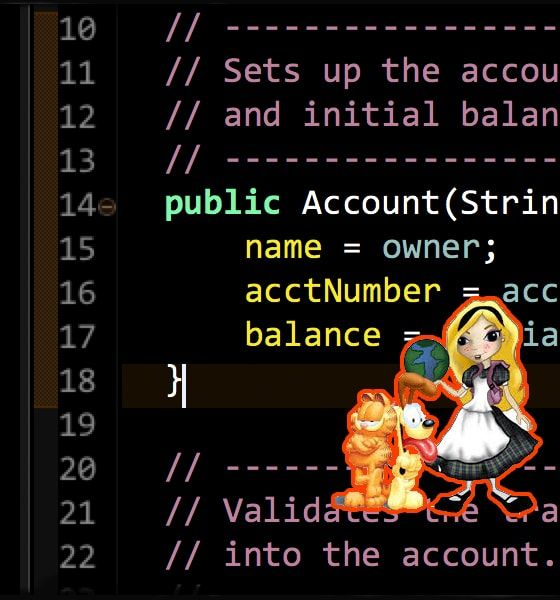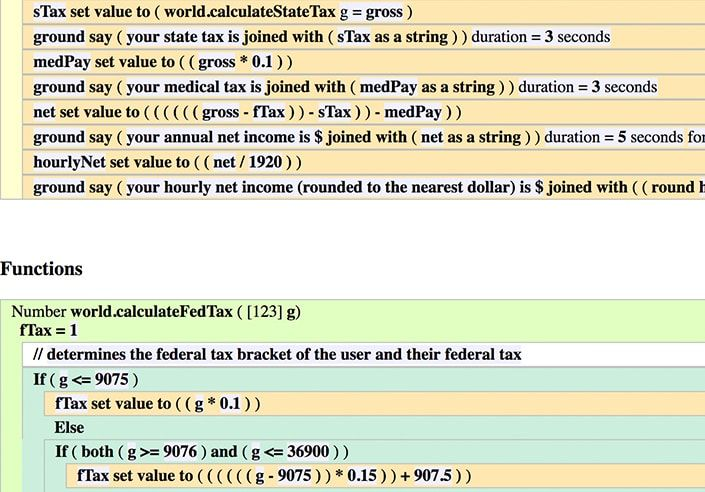Introduction to Object Oriented Programming(Java)

• Grade: 8th - 12th
• Skill Level: Beginner
• Prerequisite: None
• Software: Alice and Eclipse
• Duration: 3 Quarters. Each Quarter is 12 Weeks.

Course DescriptionIntroduction to object oriented programming using Java is the first course in computer science for the students who have no previous experience in computer science. Course is taught using project based learning approach. In this approach students develop incremental understanding of the process of problem solving and logic by experimenting, by creating engaging games and animations and by articulating patterns they observe. Introduction to OOP is taught in two phases; during the first phase students do not use syntax, focus is on developing conceptual understanding and problem solving. This is taught using the tool called Alice.Alice is an innovative 3D programming environment that makes it easy to create an animation for telling a story, or creating an interactive game, or a video to share on the web.Using this interface students drag and drop graphic tiles to create programs to solve simple problems. Instructions in these programs correspond to the statements in Object Oriented Programming like Java, C++ and C#. Second phase of this course focuses on applying concepts learnt in the previous phase and learning syntax of the Java language. This part of the course is taught using Eclipse IDE. By the end of this course students will be able to write algorithms, understand basics of computer science, use flow control in conjunctions with relational, logical and arithmetic operators to solve problems. Students will also be able to write modular programs using Java.

Film making and computer science

12 Weeks

Students are introduced to computer science by using analogies from film making. During this quarter students start to tinker with logic and problem solving while learning basics of animation and design. Students also start to write algorithms and work on dividing problems into smaller using. By the end of this quarter students are able to write functional programs.

Modular Design and Logical Structures

12 Weeks

During this quarter students gain better understanding of the use of variables, flow control and repetition structures. Students will use logical and relational operators to write modular programs and design solutions to problems. Towards the end of this quarter students start transitioning into writing code in Java.

Introduction to Java

12 Weeks

This quarter students apply the concepts they have learnt so far to write code in Java. Now that students have basic understanding of concepts focus shifts to learning syntax and structure of the Java programming language. During this quarter we cover: variables, input-output, logical structures and problem solving. This course serves as a building block to intermediate and AP Computer Science.

What will students learn?

Students will learn how to divide a bigger problem into smaller parts, this lead students to write programs that are divided into various methods and functions. Students will further understand concept of classes, objects, functions, parameters, variables, and instances. During the later part of this course students will apply these concepts to write java programs.

Goal of this course is to:

• Build Confidence
• Foster Creativity and Imagination
• Develop problem solving approach
• Apply learned concepts to write code in java.
• Help make Computer Science a tool of expression for young children.
• Understand how to divide problem into smaller parts - Modular design
• Develop concrete understanding of the fundamental concepts of computer scienceSkill Students Gain

• Program Design
• Problem solving
• Fundamentals of Java
• Computational Thinking
• Sequencing - Algorithmic Thinking
• Understanding and Application of Logical Structures.
• Dividing Problem into smaller pieces - Modular design